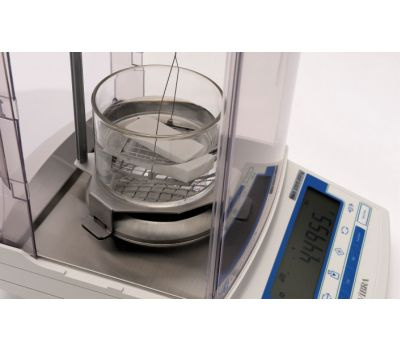Archimedes' Principle and Density DeterminationArchimedes’ Principle aids in the determination of density by providing a convenient and accurate method for determining the volume of an irregularly shaped object, like a rock. This method is quite commonly used in the construction industry. It is known also as Hydrostatic Weighing.

Example
P = Density      m = Weight or Mass in Air       m2 = Weight in Water

An object is weighed in air and found to have mass m = 500 g

It is then submerged in water and found to have apparent mass m2 = 420 g

It is clear that it has displaced mm2 = 80 g of water. Since water has a density of 1 gram/cm3, this implies:
Volume of object = m2 = 80 cm3
The density of the object is then p = mv = 50080
P = 6.25

Note that with use of a suitable “Below Balance Weighing” device, these measurements are easy to accomplish. The balance will not see the weight of the water, just the effect of the water on the object. Note! For correct results, the water should be free of contaminants and the temperature of the water is a variable that should be taken into account.